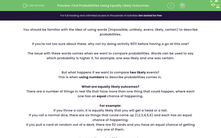# Find Probabilities Using Equally Likely Outcomes

In this worksheet, students will find probabilities using equally likely outcomes.Key stage:  KS 4

Year:  GCSE

GCSE Subjects:   Maths

GCSE Boards:   AQA, Eduqas, Pearson Edexcel, OCR,

Curriculum topic:   Probability

Curriculum subtopic:   Probability Basic Probability and Experiments

Difficulty level:#### Worksheet Overview

You should be familiar with the idea of using words (impossible, unlikely, evens, likely, certain) to describe probabilities.

If you're not too sure about these, why not try doing activity 6011 before having a go at this one?

The issue with these words comes when we want to compare probabilities. Words can be used to say which probability is higher if, for example, one was likely and one was certain.

But what happens if we want to compare two likely events?

This is when using numbers to describe probabilities comes in.

What are equally likely outcomes?

There are a number of things in real life that have more than one thing that could happen, where each one has an equal chance of happening.

For example:

If you throw a coin, it is equally likely that you will get a head or a tail.

If you roll a normal dice, there are six things that could come up (1, 2, 3, 4, 5, 6) and each has an equal chance of happening.

If you pull a card at random out of a deck, there are 52 cards and you have an equal chance of getting any one of them.

How to write probabilities as a number

There is a lovely little formula we can use here:

P (event) = Favourable outcomes ÷ total outcomes

Favourable outcomes are just the things we want to happen.

Total outcomes are the total number of things that could happen.

Example:

If I roll a normal dice, what is the chance that I get a 5?

There is only one favourable outcome here, as the number 5 only appears once.

There are six outcomes in total.

P(5 on a dice) =
 1 6

Example:

If I roll a normal dice, what is the chance I get an even number?

There are three favourable outcomes here, as there are three even numbers on a dice.

There are six outcomes in total.

P(5 on a dice) =
 3 6

Don't forget as well that you could cancel this down to make it be ½

Do probabilities have to be a fraction?

Most probabilities are given as fractions, but there's nothing wrong with changing the fractions into either a decimal or a percentage if you want to.

Let's have a go at some questions now.

### What is EdPlace?

We're your National Curriculum aligned online education content provider helping each child succeed in English, maths and science from year 1 to GCSE. With an EdPlace account you’ll be able to track and measure progress, helping each child achieve their best. We build confidence and attainment by personalising each child’s learning at a level that suits them.

Get started